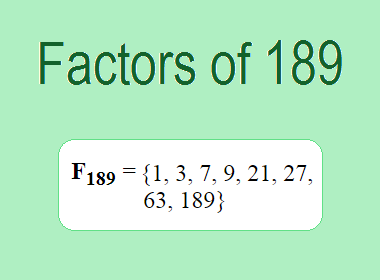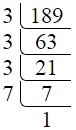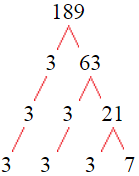# Factors of 189The factors of 189 are 1, 3, 7, 9, 21, 27, 63, and 189 i.e. F189 = {1, 3, 7, 9, 21, 27, 63, 189}. The factors of 189 are all the numbers that can divide 189 without leaving a remainder.

We can check if these numbers are factors of 189 by dividing 189 by each of them. If the result is a whole number, then the number is a factor of 189. Let's do this for each of the numbers listed above:

·        1 is a factor of 189 because 189 divided by 1 is 189.

·        3 is a factor of 189 because 189 divided by 3 is 63.

·        7 is a factor of 189 because 189 divided by 7 is 27.

·        9 is a factor of 189 because 189 divided by 9 is 21.

·        21 is a factor of 189 because 189 divided by 21 is 9.

·        27 is a factor of 189 because 189 divided by 27 is 7.

·        63 is a factor of 189 because 189 divided by 63 is 3.

·        189 is a factor of 189 because 189 divided by 189 is 1.

## How to Find Factors of 189?

1 and the number itself are the factors of every number. So, 1 and 189 are two factors of 189. To find the other factors of 189, we can start by dividing 189 by the numbers between 1 and 189. If we divide 189 by 2, we get a remainder of 1. Therefore, 2 is not a factor of 189. If we divide 189 by 3, we get a remainder of 0. Therefore, 3 is a factor of 189.

Next, we can check if 4 is a factor of 189. If we divide 189 by 4, we get a remainder of 1. Therefore, 4 is not a factor of 189. We can continue this process for all the possible factors of 189.

Through this process, we can find that the factors of 189 are 1, 3, 7, 9, 21, 27, 63, and 189. These are the only numbers that can divide 189 without leaving a remainder.

********************

********************

## Properties of the Factors of 189

The factors of 189 have some interesting properties. One of the properties is that the sum of the factors of 189 is equal to 320. We can see this by adding all the factors of 189 together:

1 + 3 + 7 + 9 + 21 + 27 + 63 + 189 = 320

Another property of the factors of 189 is that they are all odd numbers. This is because 189 is an odd number, and any even number cannot divide an odd number without leaving a remainder.

Another property of the factors of 189 is that there are only two prime factor of 189 which are 3 and 7.

## Applications of the Factors of 189

The factors of 189 have several applications in mathematics. One of the applications is in finding the highest common factor (HCF) of two or more numbers. The HCF is the largest factor that two or more numbers have in common. For example, to find the HCF of 189 and 45, we need to find the factors of both numbers and identify the largest factor they have in common. The factors of 189 are 1, 3, 7, 9, 21, 27, 63, and 189. The factors of 45 are 1, 3, 5, 9, 15, and 45. The largest factor that they have in common is 9. Therefore, the HCF of 189 and 45 is 9.

Another application of the factors of 189 is in prime factorization. Prime factorization is the process of expressing a number as the product of its prime factors. The prime factors of 189 are 3 and 7. We can express 189 as:

189 = 3 × 3 × 3 × 7

We can do prime factorization by division and factor tree method also. Here is the prime factorization of 189 by division method,189 = 3 × 3 × 3 × 7

Here is the prime factorization of 189 by the factor tree method,189 = 3 × 3 × 3 × 7

## Conclusion

The factors of 189 are the numbers that can divide 189 without leaving a remainder. The factors of 189 are 1, 3, 7, 9, 21, 27, 63, and 189. The factors of 189 have some interesting properties, such as being odd numbers and having a sum of 320. The factors of 189 have several applications in mathematics, such as finding the highest common factor and prime factorization.